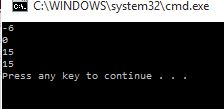# Bitwise operator in C#

##### How to use Bitwise operator in C#

Bitwise operator:

A bitwise operator is an operator used to perform bitwise operations on bit patterns or binary numerals that involve the manipulation of individual bits. The c# supports some of the following bitwise operators that are given below.

Some of the bitwise operators which we are using in this program are

1.  ~   (Not operation)

2. &    (And operation)

3.  |     (OR operation)

4.  ^   (Exclusive OR operation)

```  using System;
using System.Collections.Generic;
using System.Linq;
using System.Text;
class Program

{
static void Main()
{
int a = 5;
int b = 10;
Console.WriteLine(~a);
Console.WriteLine(a & b);
Console.WriteLine(a | b);
Console.WriteLine(a ^ b);

}
}
```

In the above code snippet we are performing bitwise operations .

1. In the first step we are performing Not opreration we have taken the a value as 5 and b value as 10 and we are performing Not operation the formula for Not operation is (-a-1)=(-5-1)=-6.

2. Second we are performing AND operation, In this the formula for AND operation is we have to convert the numbers into the decimal values 5=(0101) & 10=(1010). The AND operation calculates as (0101) & (1010) as 0 because the AND operation prints 0 value if there is 0 in the operation.

3. Next we are performing OR operation (0101) | (1010), In this operation it calculate the value as if 1 value is there in the operation it prints 1 value then  (1111)=where the binary code of (1111) is (8421) = 15, prints the value as 15,

4. Next we are performing the Exclusive OR operation, 5(0101) ^ 10(1010) as we combine the two values (0101) ^ (1010), result is  (1111), the binary code of (1111) is 15, prints the value as (15).

outputViews: 2806 | Post Order: 7